### Home > CALC > Chapter 3 > Lesson 3.2.1 > Problem3-50

3-50.
1. WHAT A DAY!
Below is a graph of the distance David traveled away from his home on a trip to the mountains. Place the events listed below in the proper order based on details from the graph.

Trace the graph below and identify the parts that correspond to each event during David's trip.
Then answer parts (a) through (d) below. Homework Help ✎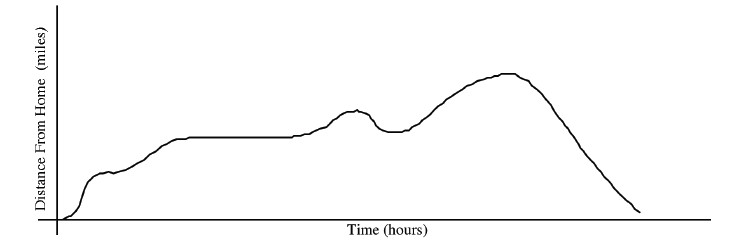EVENTS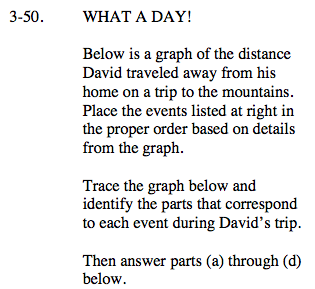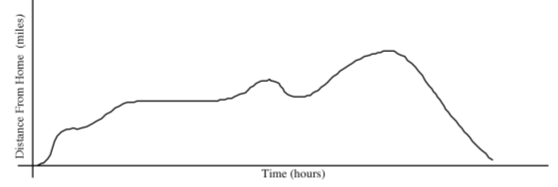The four places where events take place are the places where the slope changes:
1) The spot where the graph flattens out momentarily
2) The long horizontal section of the graph
3) Where the slope is negative for a short time
4) Where the slope is negative for a long time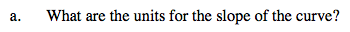$\text{slope }= \frac{\text{rise}}{\text{run}}=\frac{\Delta y}{\Delta x}= \frac{\text{units on the }y\text{ axis}}{\text{units on the }x\text{ axis}}=\underline{\ \ \ \ \ \ \ \ \ \ \ \ }$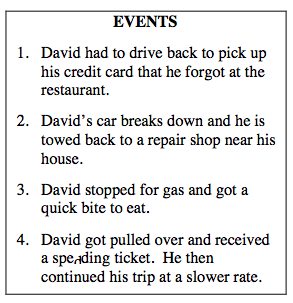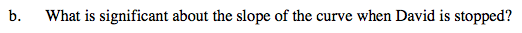moving forward → positive slope
moving backward → negative slope
stopping → __________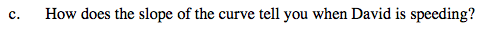If velocity is slope of the distance graph... so speeding would mean steep slope. Be mindful that speeding could mean steep-and-positive or steep-and-negative.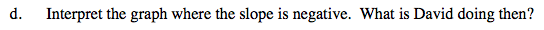Refer to the hint in part (b).